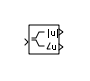Complex to Magnitude-Angle

Compute magnitude and/or phase angle of complex signal

• Library:

•Description

The Complex to Magnitude-Angle block outputs the magnitude and/or phase angle of the input signal, depending on the setting of the Output parameter. The outputs are real values of the same data type as the block input. The input can be an array of complex signals, in which case the output signals are also arrays. The magnitude signal array contains the magnitudes of the corresponding complex input elements. The angle output similarly contains the angles of the input elements.

Ports

Input

expand all

Complex input signal that the block computes and outputs the magnitude and/or the phase angle.

Data Types: single | double

Output

expand all

Output signal that is the magnitude and/or phase angle of the input signal. To choose the output, set the Output parameter.

Data Types: single | double

Parameters

expand all

Specify if the output is the magnitude and/or the phase angle in radians of the input signal.

Command-Line Information

 Parameter: Output Type: character vector Values: 'Magnitude and angle' | 'Magnitude' | 'Angle' Default: 'Magnitude and angle'

Specify the sample time as a value other than -1. For more information, see Specify Sample Time.

Dependencies

This parameter is not visible unless it is explicitly set to a value other than -1. To learn more, see Blocks for Which Sample Time Is Not Recommended.

Programmatic Use

 Block Parameter: SampleTime Type: character vector Values: scalar or vector Default: '-1'

Block Characteristics

 Data Types double | single Direct Feedthrough yes Multidimensional Signals yes Variable-Size Signals yes Zero-Crossing Detection no